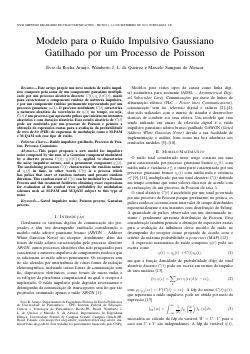##### Modelo para o Ruído Impulsivo Gaussiano Gatilhado por um Processo de Poisson

###### Abstract
This paper proposes a new model for impulsive noise composed by the sum of a Gaussian component modulated by a discrete process C(t), ηi(t)C(t), applied to characterize the noisy impulsive nature, and a permanent component ηg(t). The modulating processes C(t) characterizes the random nature of ηi(t) in time, in other words C(t) is a process which has pulses that start at random instants and present random duration. This random state of C(t) can be modeled by a Poisson Process and this treatment facilitates obtaining exact expressions for evaluation of the symbol error probability for modulation schemes such as M-PAM and M-QAM subject to this type of noise.